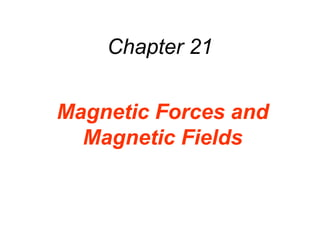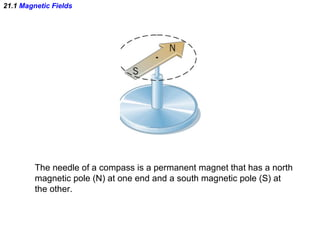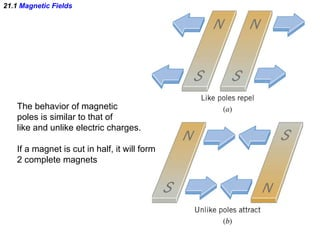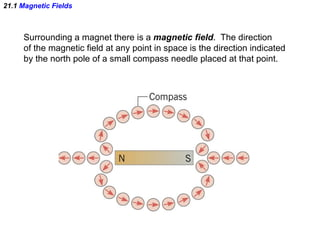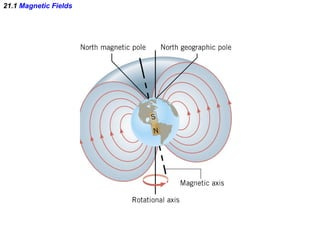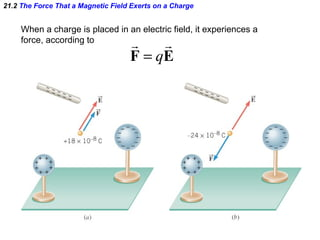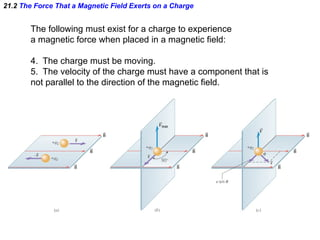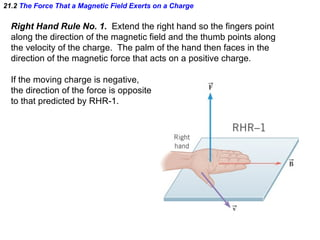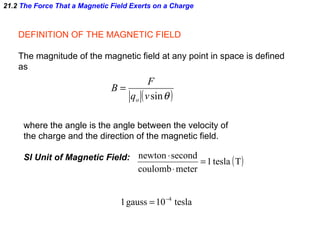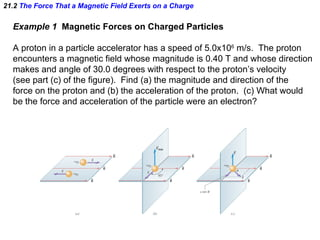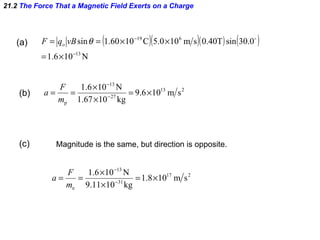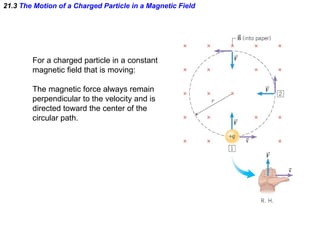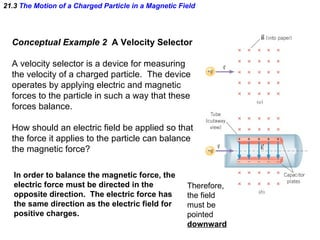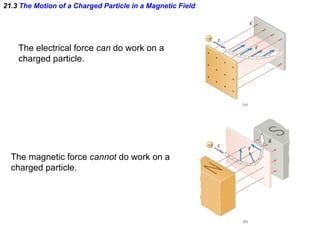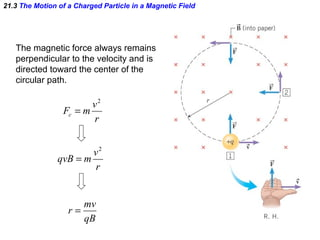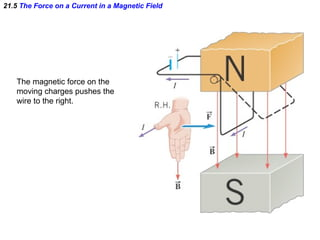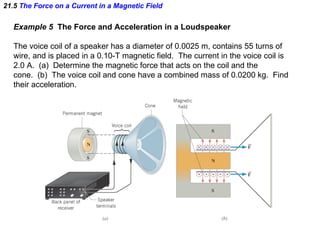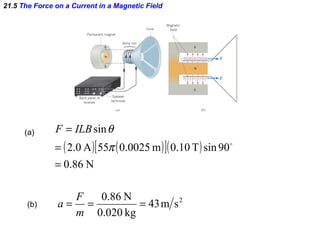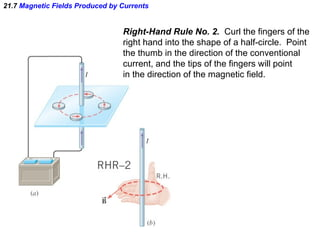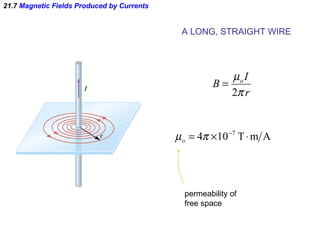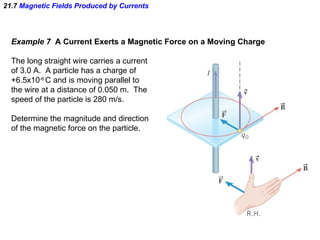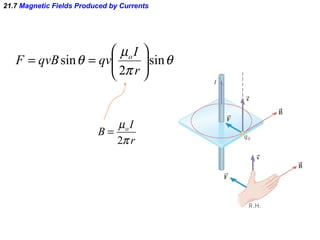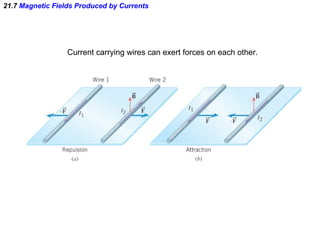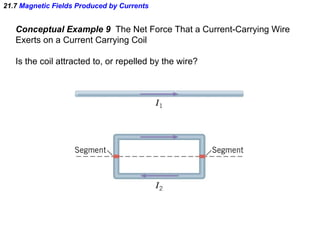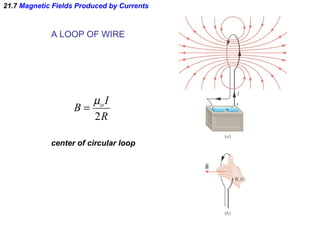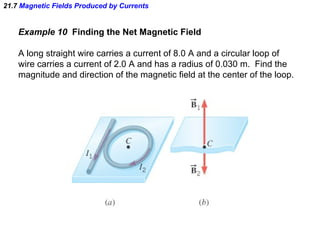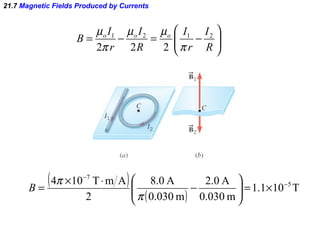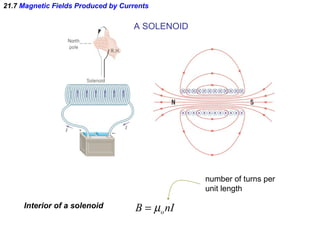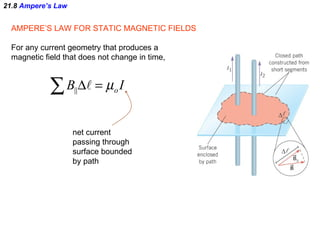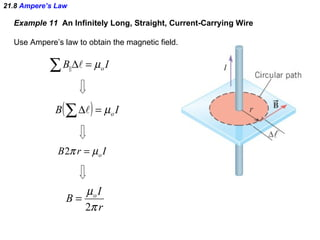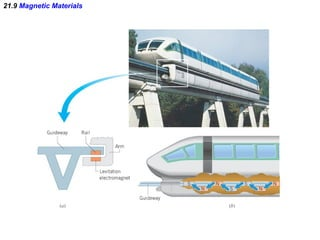1 of 31

### AP Physics - Chapter 21 Powerpoint

1. Chapter 21 Magnetic Forces and Magnetic Fields
2. 21.1 Magnetic Fields The needle of a compass is a permanent magnet that has a north magnetic pole (N) at one end and a south magnetic pole (S) at the other.
3. 21.1 Magnetic Fields The behavior of magnetic poles is similar to that of like and unlike electric charges. If a magnet is cut in half, it will form 2 complete magnets
4. 21.1 Magnetic Fields Surrounding a magnet there is a magnetic field . The direction of the magnetic field at any point in space is the direction indicated by the north pole of a small compass needle placed at that point.
5. 21.1 Magnetic Fields
6. 21.2 The Force That a Magnetic Field Exerts on a Charge When a charge is placed in an electric field, it experiences a force, according to
7. 21.2 The Force That a Magnetic Field Exerts on a Charge Right Hand Rule No. 1. Extend the right hand so the fingers point along the direction of the magnetic field and the thumb points along the velocity of the charge. The palm of the hand then faces in the direction of the magnetic force that acts on a positive charge. If the moving charge is negative, the direction of the force is opposite to that predicted by RHR-1.
8. 21.2 The Force That a Magnetic Field Exerts on a Charge DEFINITION OF THE MAGNETIC FIELD The magnitude of the magnetic field at any point in space is defined as where the angle is the angle between the velocity of the charge and the direction of the magnetic field. SI Unit of Magnetic Field:
9. 21.2 The Force That a Magnetic Field Exerts on a Charge Example 1 Magnetic Forces on Charged Particles A proton in a particle accelerator has a speed of 5.0x10 6 m/s. The proton encounters a magnetic field whose magnitude is 0.40 T and whose direction makes and angle of 30.0 degrees with respect to the proton’s velocity (see part (c) of the figure). Find (a) the magnitude and direction of the force on the proton and (b) the acceleration of the proton. (c) What would be the force and acceleration of the particle were an electron?
10. 21.2 The Force That a Magnetic Field Exerts on a Charge (a) (b) (c) Magnitude is the same, but direction is opposite.
11. 21.3 The Motion of a Charged Particle in a Magnetic Field For a charged particle in a constant magnetic field that is moving: The magnetic force always remain perpendicular to the velocity and is directed toward the center of the circular path.
12. 21.3 The Motion of a Charged Particle in a Magnetic Field Conceptual Example 2 A Velocity Selector A velocity selector is a device for measuring the velocity of a charged particle. The device operates by applying electric and magnetic forces to the particle in such a way that these forces balance. How should an electric field be applied so that the force it applies to the particle can balance the magnetic force? In order to balance the magnetic force, the electric force must be directed in the opposite direction. The electric force has the same direction as the electric field for positive charges. Therefore, the field must be pointed downward
13. 21.3 The Motion of a Charged Particle in a Magnetic Field The electrical force can do work on a charged particle. The magnetic force cannot do work on a charged particle.
14. 21.3 The Motion of a Charged Particle in a Magnetic Field The magnetic force always remains perpendicular to the velocity and is directed toward the center of the circular path.
15. 21.5 The Force on a Current in a Magnetic Field The magnetic force on the moving charges pushes the wire to the right.
16. 21.5 The Force on a Current in a Magnetic Field Example 5 The Force and Acceleration in a Loudspeaker The voice coil of a speaker has a diameter of 0.0025 m, contains 55 turns of wire, and is placed in a 0.10-T magnetic field. The current in the voice coil is 2.0 A. (a) Determine the magnetic force that acts on the coil and the cone. (b) The voice coil and cone have a combined mass of 0.0200 kg. Find their acceleration.
17. 21.5 The Force on a Current in a Magnetic Field (a) (b)
18. 21.7 Magnetic Fields Produced by Currents Right-Hand Rule No. 2. Curl the fingers of the right hand into the shape of a half-circle. Point the thumb in the direction of the conventional current, and the tips of the fingers will point in the direction of the magnetic field.
19. 21.7 Magnetic Fields Produced by Currents A LONG, STRAIGHT WIRE permeability of free space
20. 21.7 Magnetic Fields Produced by Currents Example 7 A Current Exerts a Magnetic Force on a Moving Charge The long straight wire carries a current of 3.0 A. A particle has a charge of +6.5x10 -6 C and is moving parallel to the wire at a distance of 0.050 m. The speed of the particle is 280 m/s. Determine the magnitude and direction of the magnetic force on the particle.
21. 21.7 Magnetic Fields Produced by Currents
22. 21.7 Magnetic Fields Produced by Currents Current carrying wires can exert forces on each other.
23. 21.7 Magnetic Fields Produced by Currents Conceptual Example 9 The Net Force That a Current-Carrying Wire Exerts on a Current Carrying Coil Is the coil attracted to, or repelled by the wire?
24. 21.7 Magnetic Fields Produced by Currents A LOOP OF WIRE center of circular loop
25. 21.7 Magnetic Fields Produced by Currents Example 10 Finding the Net Magnetic Field A long straight wire carries a current of 8.0 A and a circular loop of wire carries a current of 2.0 A and has a radius of 0.030 m. Find the magnitude and direction of the magnetic field at the center of the loop.
26. 21.7 Magnetic Fields Produced by Currents
27. 21.7 Magnetic Fields Produced by Currents A SOLENOID Interior of a solenoid number of turns per unit length
28. 21.8 Ampere’s Law AMPERE’S LAW FOR STATIC MAGNETIC FIELDS For any current geometry that produces a magnetic field that does not change in time, net current passing through surface bounded by path
29. 21.8 Ampere’s Law Example 11 An Infinitely Long, Straight, Current-Carrying Wire Use Ampere’s law to obtain the magnetic field.
30. 21.9 Magnetic Materials